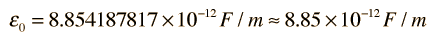Back to Course

0% Complete
0/162 Steps

• ## Recent Posts

• #### Daily Analysis: 17th October 2020

In Progress
Lesson 2, Topic 1
In Progress

# Electric Field Due to a Point Charge

Lesson Progress
0% Complete

Electric field is defined as the electric force per unit charge. The direction of the field is taken to be the direction of the force it would exert on a positive test charge. The electric field is radially outward from a positive charge and radially in toward a negative point charge.Click on any of the examples above for more detail.

The electric field from any number of point charges can be obtained from a vector sum of the individual fields. A positive number is taken to be an outward field; the field of a negative charge is toward it.

This electric field expression can also be obtained by applying Gauss’ law.

In the equations describing electric and magnetic fields and their propagation, three constants are normally used. One is the speed of light c, and the other two are the electric permittivity of free space ?0 and the magnetic permeability of free space, ?0. The magnetic permeability of free space is taken to have the exact value

This contains the force unit N for Newton and the unit A is the Ampere, the unit of electric current.

With the magnetic permeability established, the electric permittivity takes the value given by the relationshipwhere the speed of light c is given byThis gives a value of free space permittivitywhich in practice is often used in the formThese expressions contain the units F for Farad, the unit of capacitance, and C for Coulomb, the unit of electric charge.

In the presence of polarizable or magnetic media, the effective constants will have different values. In the case of a polarizable medium, called a dielectric, the comparison is stated as a relative permittivity or a dielectric constant. In the case of magnetic media, the relative permeability may be stated.

Expressions for the electric and magnetic fields in free space contain the electric permittivity ?0 and magnetic permeability ?0 of free space. As indicated in the section on electric and magnetic constants, these two quantities are not independent but are related to “c”, the speed of light and other electromagnetic waves.